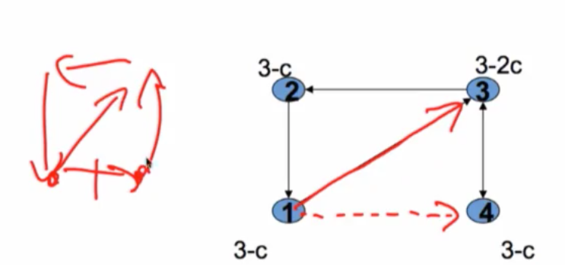# Directed Networks¶

• Formation game easy

• Players simutaneously announce their preferred set of neighbors $S_i$

• g(S) = {ij : j in $S_i$} keeping track of ordered pairs

• Nash equilibrium

# Flow of Payoffs?¶

• One way flow - get information but not vice versa

• Two way flow - one player bears the cost, but both benefit from the connection (link on internet, phone call??)

# Two Way Flow¶

• Efficiency as in the undirected connections model, except c/2 and link in either direction (but not both)

• we have half as much costs being born, so instead of having 2c per link, we're going to have 1c per link, and then in term of what that does for the efficient calculations, is it just factors everything down by a factor of 2 in terms of the costs. So the efficiency in this model is exactly as it was before except for we've divided through by 2
• low cost: $c/2 < \delta - \delta^2$ ==> "complete" networks, "complete" in quotes, because complete doesn't mean that every link in both directions is present, it just means that every two nodes are connected, and in this case, they're connected by only one link. It doesn't make sense to have links in both directions because you bear twice the cost and there's no added information flow in this situation.
• medium cost: $\delta - \delta^2 < c/2 <\delta + (n - 2)\delta^2/2$ ==> "star" networks
• high cost: $\delta + (n - 2)\delta^2/2 < c/2$ ==> empty network
• Nash Stable:

• low cost: $c < \delta - \delta^2$ ==> two-way "complete" networks are Nash stable
• medium/low cost: $\delta - \delta^2 <c <\delta$ ==> all star networks are Nash stable, plus others
• medium/high cost: $\delta <c < \delta + (n-2)\delta^2/2$ ==> peripherally sponsored star networks are Nash stable(no other stars, but sometimes other networks)
• $\delta - \delta^2 <c < 2(\delta - \delta^2)$ complete is efficient, not equilibrium

# One Way Flow¶

• Keep track of directed flows, and in links are not (always) useful

# An Example¶

• Bala and Goyal(00) - Directed connections model with no decay

• $u_i(g) = R_i(g) - d_i^{out}(g)c$

• where $R_i(g)$ is the number of players reached by directed paths from i

# Efficient Networks¶

• n‐player "wheels" if c< n‐1, empty otherwise:# Stable Networks¶

• if c<1 then n-player wheels are the only strictly Nash stable network
• if 1<c<n-1 n-player wheels and empty networks are the only strictly Nash stable networks

# Strictness¶

• Nash Stable, but not strictly so: 1 is indifferent between switching link from 3 to 4